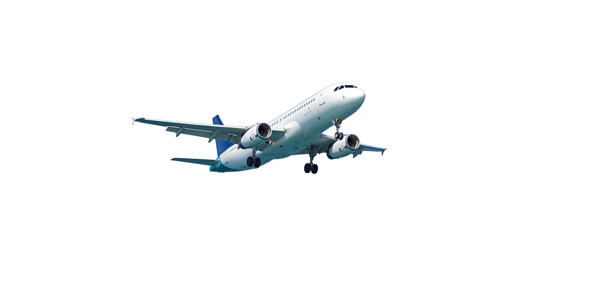# CDC 2a651s Volume 2 By CDCmaster

88 Questions | Total Attempts: 92Settings.

• 1.
An aircraft taxiing at a steady speed can be used to demonstrate
• A.

Bernoulli's principle

• B.

Newton's first law of motion

• C.

Newton's second law of motion

• D.

Newton's third law of motion

• 2.
Newton's third law of motion explains why an operating jet engine produces
• A.

Forward thrust

• B.

Forward acceleration

• C.

Increased acceleration

• D.

Decreased acceleration

• 3.
Which type of duct would decrease the velocity and increase the pressure of a gas as it passes through?
• A.

Elbow

• B.

Straight

• C.

Divergent

• D.

Convergent

• 4.
"The combination of decreased pressure above an airfoil, and the increased pressure below the airfoil produces lift" is best described by
• A.

Bernoulli's principle

• B.

Newton's first law of motion

• C.

Newton's second law of motion

• D.

Newton's third law of motion

• 5.
Foot-pounds and inch-pounds are units of measure for
• A.

Work

• B.

Power

• C.

Inertia

• D.

Friction

• 6.
What does fuel for an engine represent?
• A.

Available horsepower

• B.

Thrust produced

• C.

Potential energy

• D.

Efficiency ratio

• 7.
Which section of a jet engine continually supplies air and maintains pressure?
• A.

Turbine

• B.

Diffuser

• C.

Compressor

• D.

Combustion

• 8.
The temperature of compressed air in a jet engine must be raised to
• A.

Increase energy

• B.

Decrease energy

• C.

Increase volume

• D.

Decrease volume

• 9.
Which section of a jet engine introduces and burns fuel?
• A.

Turbine

• B.

Diffuser

• C.

Compressor

• D.

Combustion

• 10.
How many British thermal units (Btu) does the heat content per pound of kerosene produce?
• A.

10500

• B.

15000

• C.

18500

• D.

40000

• 11.
The efficiency of a jet engine is often referred to as
• A.

Energy

• B.

Momentum

• C.

Thermal efficiency

• D.

Propulsive efficiency

• 12.
The product of thermal efficiency and propulsive efficiency is
• A.

Mechanical efficiency

• B.

Overall efficiency

• C.

Power efficiency

• D.

Thrust efficiency

• 13.
To measure barometric pressure, you would use a
• A.

Potentiometer

• B.

Psychrometer

• C.

Pyrometer

• D.

Barometer

• 14.
What effect does moisture content or humidity have on density of air?
• A.

More moisture, more dense

• B.

More moisture, less dense

• C.

Less moisture, less dense

• D.

None

• 15.
Due to the divergent design of a diffuser, air pressure
• A.

Remains the same

• B.

Fluctuates

• C.

Decreases

• D.

Increases

• 16.
The air temperature of a jet engine gradually rises across the compressor to the diffuser outlet as a result of
• A.

Compression

• B.

Fuel expansion

• C.

Turbine discharge

• D.

Ambient temperature increase

• 17.
Where is the highest point of temperature reached in an engine?
• A.

Tailpipe

• B.

Exhaust cone

• C.

Turbine section

• D.

Combustion section

• 18.
Which engine component meters fuel for combustion?
• A.

Pressure and drain (P&D) valve

• B.

Fuel control

• C.

Fuel nozzle

• D.

Fuel pump

• 19.
What happens to the energy that is absorbed by the turbine wheel?
• A.

50 percent is used for accessories

• B.

60 percent is used for accessories

• C.

It is returned to the compressor

• D.

It operates the anti-ice system

• 20.
What is the required velocity for air and gases flowing through a jet engine?
• A.

Low velocity at all times

• B.

Less velocity exiting than entering

• C.

Equal velocity entering and exiting

• D.

Greater velocity exiting than entering

• 21.
The velocity of the stream of air that flows through a jet engine reaches its highest point at the
• A.

Ejector nozzle

• B.

Combustion section

• C.

Turbine exhaust cone

• D.

Turbine stator (nozzle diaphragm)

• 22.
What is the general flow of gases from the combustion chamber?
• A.

At right angles to the rotor shaft

• B.

Parallel to the axis of the rotor shaft

• C.

At the same velocity as the rotor shaft

• D.

In a reverse direction from the rotor shaft

• 23.
Gases enter the jet-engine's first-stage turbine wheel blades from the
• A.

• B.

Combustion chamber

• C.

Turbine stator

• D.

Flameholder

• 24.
When two or more turbine wheels are used in a jet engine, which component is placed directly in front of each turbine wheel?
• A.

Diffuser

• B.

Jet nozzle

• C.

Combustion chamber

• D.

Turbine stator (nozzle diaphragm)

• 25.
A jet engine derives its name from its design in that it uses
• A.

An exhaust-gas-driven turbine wheel to drive its compressor

• B.

Nozzles which are called jet within the engine

• C.

A turbine-type compressor to maintain power

• D.

Turbo-superchargers within the engine

Related TopicsBack to top